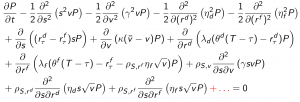Figure: 4 dimensional (Kolmogorov-forward) probability density P of one single FX rate

The research article “Efficient exposure computation by risk factor decomposition” by Kees de Graaf, Drona Kandhai and Christoph Reisinger was published in Quantitative Finance.

The focus of this paper is the efficient computation of counterparty credit risk exposure on portfolio level. Here, the large number of risk factors rules out traditional PDE-based techniques and allows only a relatively small number of paths for nested Monte Carlo simulations, resulting in large variances of estimators in practice. We propose a novel approach based on Kolmogorov forward and backward PDEs, where we counter the high dimensionality by a generalisation of anchored-ANOVA decompositions. By computing only the most significant terms in the decomposition, the dimensionality is reduced effectively, such that a significant computational speed-up arises from the high accuracy of PDE schemes in low dimensions compared to Monte Carlo estimation. Moreover, we show how this truncated decomposition can be used as control variate for the full high-dimensional model, such that any approximation errors can be corrected while a substantial variance reduction is achieved compared to the standard simulation approach. We investigate the accuracy for a realistic portfolio of exchange options, interest rate and cross-currency swaps under a fully calibrated ten-factor model.